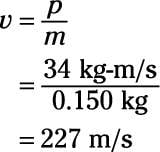##### Physics I Workbook For Dummies with Online Practice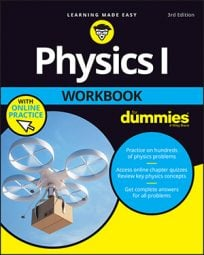It turns out that there’s a direct connection between impulse and momentum. If you hit a pool ball with a cue, the cue imparts a certain impulse to the ball, causing the ball to end up with a particular momentum.

How can you relate impulse to momentum? Easy. The impulse you impart to an object gives it a change in momentum equal to that impulse, so

You might be confused about the units here. Impulse has MKS units of N-s, but momentum has MKS units of kg-m/s. If the units are different, then how can these two be set equal to each other? Fortunately, the units are not actually different here. Recall that 1 N = 1 kg-m/s2. This means that 1 N-s = 1 kg-m/s.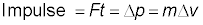## Sample question

1. If you hit a stationary 160.0-g pool ball with a force of 100.0 N for 0.10 seconds, what is its final speed?

The correct answer is 62 m/s.

1. Use the equation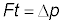2. Find the impulse first:

Impulse = Ft = (100.0 N)(0.10 s) = 10 N-s

3. That impulse is the change in the pool ball’s momentum: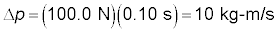4. Now the pool ball’s initial momentum was zero, so the final momentum is: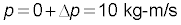5. Momentum equals mass times velocity, so to find speed (the magnitude of velocity), you can solve for v, like so: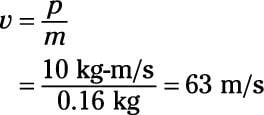## Practice questions

1. You hit a hockey puck, mass 170 g, with a force of 150 N for 0.10 seconds. If it started at rest, what is its final speed?

2. You’re standing on an ice rink when another skater hits you, imparting a force of 200.0 N for 0.20 seconds. If you have a mass of 90.0 kg, what is your final speed?

3. You kick a 450-g soccer ball with a force of 400.0 N for 0.20 seconds. What is its final speed?

4. You hit a 150-g baseball with a force of 400.0 N for 0.10 seconds. The baseball was traveling toward you at 40 m/s. What is its final speed?

Following are answers to the practice questions:

1. 88 m/s

1. Use the equation2. Find the impulse:

Impulse = Ft = (150 N)(0.10 s) = 15 N-s

3. Because the initial momentum of the puck was zero, that impulse becomes the puck’s new momentum:

p = (150 N)(0.10 s) = 15 kg-m/s

4. Momentum equals mass times velocity, so to find speed (the magnitude of velocity), you solve for v: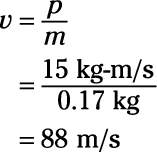2. 0.44 m/s

1. Use the equation2. Find the impulse:

Impulse = Ft = (200.0 N)(0.20 s) = 40 N-s

3. Because your initial momentum was zero, that impulse becomes your new momentum:

p = (200.0 N)(0.20 s) = 40 kg-m/s

4. Momentum equals mass times velocity, so to find speed (the magnitude of velocity), you solve for v: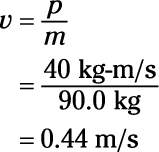3. 178 m/s

1. Use the equation2. 2.Find the impulse:

Impulse = Ft = (400.0 N)(0.20 s) = 80 N-s

3. That impulse becomes the soccer ball’s new momentum:

p = (400.0 N)(0.20 s) = 80 kg-m/s

4. Momentum equals mass times velocity, so to find speed (the magnitude of velocity), you solve for v: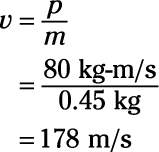4. 227 m/s

1. Use the equation2. Find the impulse first:

Impulse = Ft = (400.0 N)(0.10 s) = 40 N-s

3. That impulse becomes the ball’s change in momentum: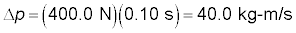4. The baseball started with a momentum of (0.150 kg)(40 m/s) = 6 kg-m/s (toward you), so its final momentum will be 40 kg-m/s – 6 kg-m/s = 34 kg-m/s (away from you).

5. Momentum equals mass times velocity, so to find speed (the magnitude of velocity), you solve for v: